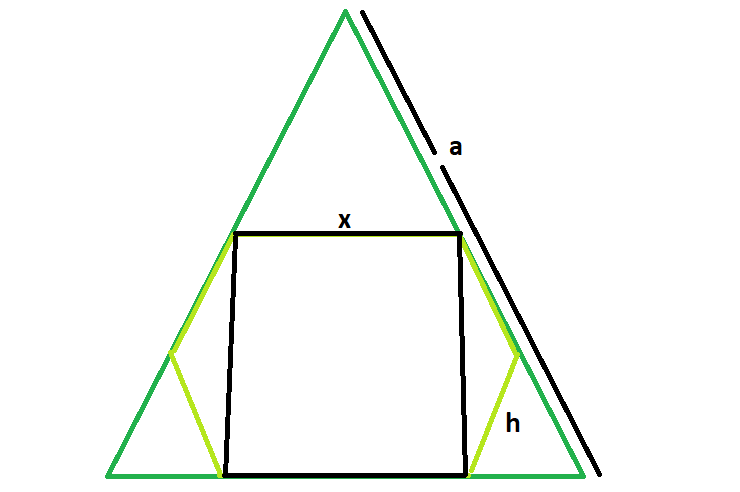# Largest square that can be inscribed within a hexagon which is inscribed within an equilateral triangle

Given here is an equilateral triangle of side length a, which inscribes a hexagon which in turn inscribes a square. The task is to find the side length of the square.

Examples:

```Input:  a = 6
Output: 2.538

Input: a = 8
Output: 3.384
```## Recommended: Please try your approach on {IDE} first, before moving on to the solution.

Approach:
We know the, side length of a hexagon inscribed within an equilateral triangle is h = a/3. Please refer Largest hexagon that can be inscribed within an equilateral triangle .

Also, the side length of the square that can be inscribed within a hexagon is x = 1.268h Please refer Largest Square that can be inscribed within a hexagon.

So, side length of the square inscribed within a hexagon which in turn is inscribed within an equilateral triangle, x = 0.423a.

Below is the implementation of the above approach:

## C++

 `// C++ program to find the side of the largest square ` `// that can be inscribed within the hexagon which in return ` `// is incsribed within an equilateral triangle ` `#include ` `using` `namespace` `std; ` ` `  `// Function to find the side ` `// of the square ` `float` `squareSide(``float` `a) ` `{ ` ` `  `    ``// Side cannot be negative ` `    ``if` `(a < 0) ` `        ``return` `-1; ` ` `  `    ``// side of the square ` `    ``float` `x = 0.423 * a; ` `    ``return` `x; ` `} ` ` `  `// Driver code ` `int` `main() ` `{ ` `    ``float` `a = 8; ` `    ``cout << squareSide(a) << endl; ` ` `  `    ``return` `0; ` `} `

## Java

 `// Java program to find the side of the ` `// largest square that can be inscribed  ` `// within the hexagon which in return is  ` `// incsribed within an equilateral triangle ` `class` `cfg  ` `{ ` `     `  `// Function to find the side ` `// of the square ` `static` `float` `squareSide(``float` `a) ` `{ ` ` `  `    ``// Side cannot be negative ` `    ``if` `(a < ``0``) ` `        ``return` `-``1``; ` ` `  `    ``// side of the square ` `    ``float` `x = (``0``.423f * a); ` `    ``return` `x; ` `} ` ` `  `// Driver code ` `public` `static` `void` `main(String[] args) ` `{ ` `    ``float` `a = ``8``; ` `    ``System.out.println(squareSide(a)); ` ` `  `} ` `} ` ` `  `// This code is contributed by  ` `// Mukul Singh. `

## Python3

 `# Python 3 program to find the side of the  ` `# largest square that can be inscribed  ` `# within the hexagon which in return ` `# is incsribed within an equilateral triangle ` ` `  `# Function to find the side of the square ` `def` `squareSide(a): ` `     `  `    ``# Side cannot be negative ` `    ``if` `(a < ``0``): ` `        ``return` `-``1` ` `  `    ``# side of the square ` `    ``x ``=` `0.423` `*` `a ` `    ``return` `x ` ` `  `# Driver code ` `if` `__name__ ``=``=` `'__main__'``: ` `    ``a ``=` `8` `    ``print``(squareSide(a)) ` ` `  `# This code is contributed by ` `# Sanjit_Prasad `

## C#

 `// C# program to find the side of the ` `// largest square that can be inscribed  ` `// within the hexagon which in return is  ` `// incsribed within an equilateral triangle ` `using` `System; ` ` `  `class` `GFG ` `{ ` `     `  `// Function to find the side ` `// of the square ` `static` `float` `squareSide(``float` `a) ` `{ ` ` `  `    ``// Side cannot be negative ` `    ``if` `(a < 0) ` `        ``return` `-1; ` ` `  `    ``// side of the square ` `    ``float` `x = (0.423f * a); ` `    ``return` `x; ` `} ` ` `  `// Driver code ` `public` `static` `void` `Main() ` `{ ` `    ``float` `a = 8; ` `    ``Console.WriteLine(squareSide(a)); ` `} ` `} ` ` `  `// This code is contributed by  ` `// shs `

## PHP

 ` `

Output:

```3.384
```

GeeksforGeeks has prepared a complete interview preparation course with premium videos, theory, practice problems, TA support and many more features. Please refer Placement 100 for details

My Personal Notes arrow_drop_upCheck out this Author's contributed articles.

If you like GeeksforGeeks and would like to contribute, you can also write an article using contribute.geeksforgeeks.org or mail your article to contribute@geeksforgeeks.org. See your article appearing on the GeeksforGeeks main page and help other Geeks.

Please Improve this article if you find anything incorrect by clicking on the "Improve Article" button below.